1593459240

# Geometric Deep Learning: A Quick Tour

The following document provides a whirlwind tour of some fundamental concepts in geometric deep learning. The mathematical derivations might not be as rigorously shown and some equations are stated without proofs. This is done intentionally to keep the document short yet comprehensive enough. For deeper analysis, please refer to Bronstein et al. (2017), Gong (2018), Kipf et al. (2017), Hammond et al. (2011), Shuman et al. (2011) and . This is a living document, so please let me know if you find any errors or inconsistency, I will fix them as soon as possible. I am by no means an expert in this field as this note is merely written for my own learning purpose.

We first start by reviewing the concept of a Laplacian matrix in a graph and its’ eigenvalue decomposition. Next, we define convolution operation in the graph and show that it is equivalent to applying a filter in the spectral domain of the graph, where spectrum here refers to the eigenvalues of the laplacian. We show that spectral convolution, by construction, is similar to applying Laplacian operator to function. Lastly, we show how to approximate the Laplacian filter with the sum of Chebyshev polynomials to reduce the algorithm complexity (as performing the complete eigenvalue decomposition is O(n²) where n is the number of vertices in the graph.

Graph Laplacian

Assume we have an undirected, connected and weighted graph G = (V, E, W) where V is a set of |V| = n vertices, **E **is a set of edges and W is a set of weights _W_ᵢⱼ for each edge i~j. Define D as the degree matrix where D = diag(Σⱼ _W_ᵢⱼ). The normalized graph laplacian Δ can be defined as the following.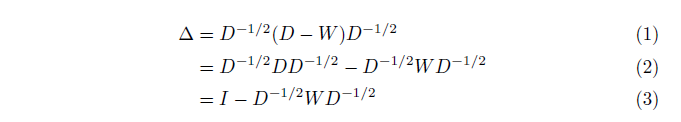We can perform eigenvalue decomposition to the laplacian matrix Δ as the following.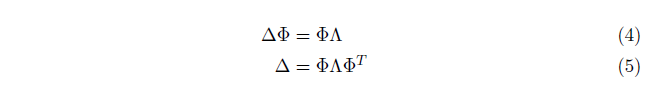# Spectral Convolution

Motivated by the convolution operation in Fourier Transform, we define graph convolution as applying a filter to the laplacian spectral components. In a nutshell, the idea is to project the input function f to its’ “Fourier” basis, or here laplacian eigenvectors by means of Φᵀ** f**. Multiply the basis with filtered eigenvalues ĥ(Λ), resulting in ĥ(Λ) Φᵀ** f**. Lastly, apply an “inverse Fourier transform” by dot product with Φ, resulting in Φ ĥ(Λ) Φᵀ** f**.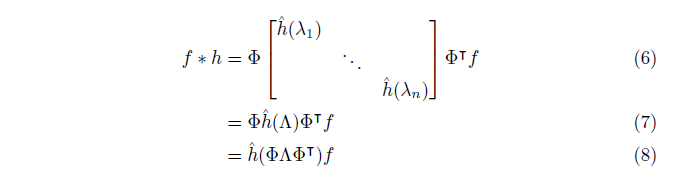After applying some rearrangements, we can see that the right hand side of the equation can be converted to a function of the normalized laplacian matrix Δ = Φ Λ Φᵀ from equation 5.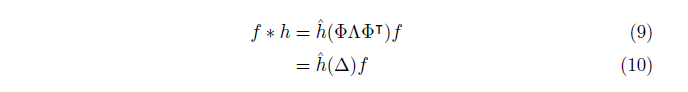Now, similar to a convolutional neural network, we would like to apply more than 1 convolutional filters and also add a nonlinear output transformation. Suppose we use p filters with ξ nonlinear transformation, the spectral convolution network is defined as the following.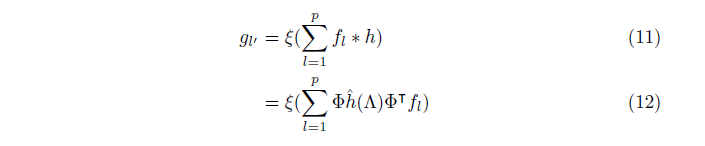Approximation with Chebyshev Polynomials

## ChebNets

The main problem with equation 12 is that we need to calculate all eigenvectors which is O(|V|²) in complexity. This is not scalable when we deal with a very large graph with |V|>>. The main idea in ChebNets is to approximate the Φ ĥ(Λ) Φᵀ with a sum of orthonormal Chebyshev polynomials that can be calculated iteratively using the following formula.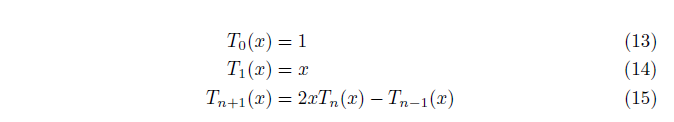We first approximate the eigenvalue filter with a weighted sum of r Chebyshev polynomials that takes in the transformed eigenvalue matrix Λ^. This transformation is needed because Chebyshev polynomials form an orthogonal basis only when the input domain interval is in [-1, 1]. Meanwhile, the eigenvalues range from [0, λₘₐₓ**]. Hence to transform the latter to the former, we can apply 2x/λₘₐₓ -1** such that for x = 0, (2(0)/λₘₐₓ-1 = -1) and for x = λₘₐₓ, (2λₘₐₓ/λₘₐₓ -1 = 1).We can now take equation 12 and substitute the filter with its’ Chebyshev polynomials. Note that Λ^ = 2 Λ / λₘₐₓ - I and similarly, Δ^ = 2 Δ/ λₘₐₓ - I. By means of rearrangement, we can transform the right hand side into a sum of Chebyshev polynomials applied on the normalized laplacian Δ^.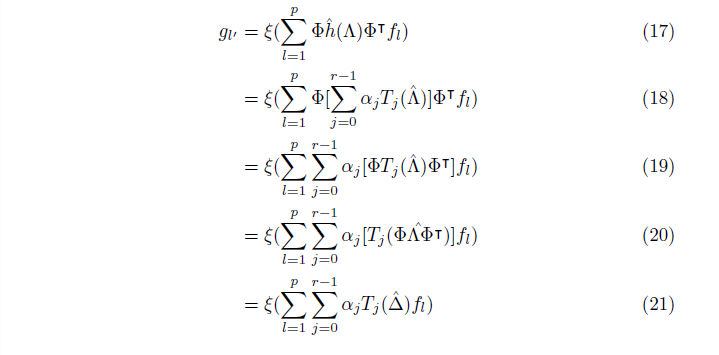#convolutional-network #data-science #mathematics #geometric-deep-learning #deep-learning #deep learning

## Buddha Community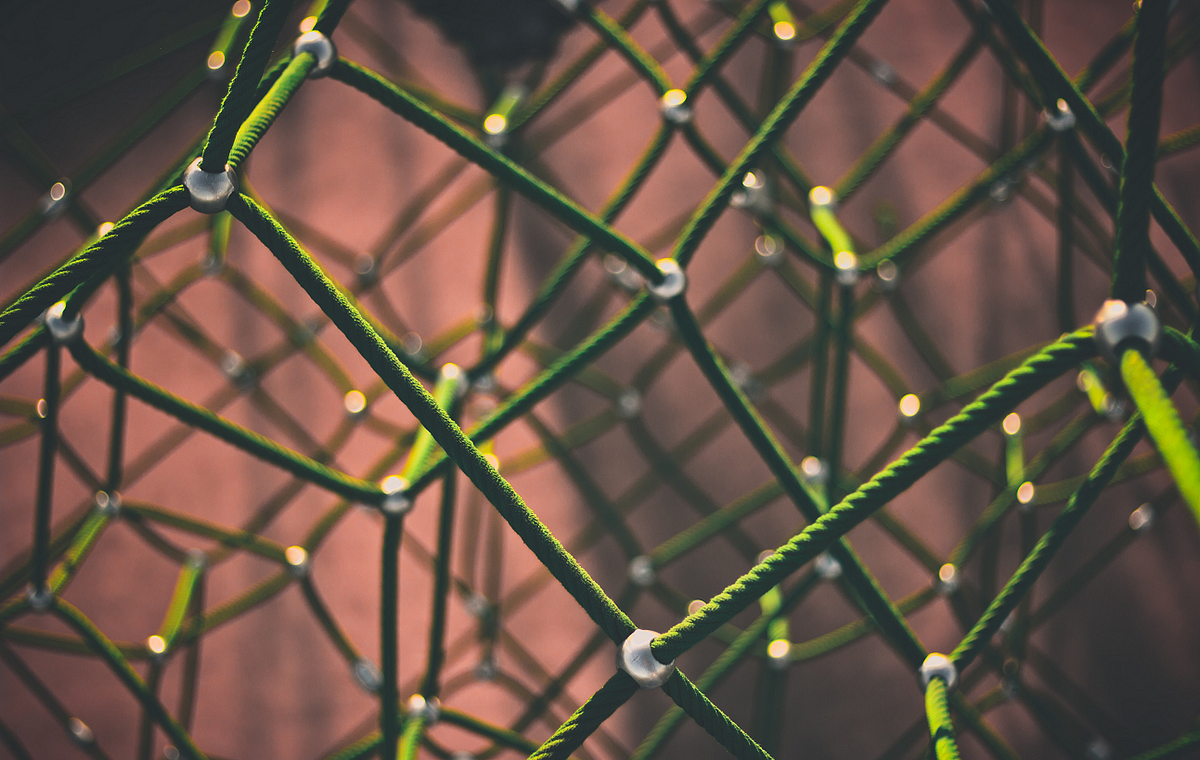1618317562

## Top Deep Learning Development Services | Hire Deep Learning Developer

We at Inexture, strategically work on every project we are associated with. We propose a robust set of AI, ML, and DL consulting services. Our virtuoso team of data scientists and developers meticulously work on every project and add a personalized touch to it. Because we keep our clientele aware of everything being done associated with their project so there’s a sense of transparency being maintained. Leverage our services for your next AI project for end-to-end optimum services.

#deep learning development #deep learning framework #deep learning expert #deep learning ai #deep learning services1593459240

## Geometric Deep Learning: A Quick Tour

The following document provides a whirlwind tour of some fundamental concepts in geometric deep learning. The mathematical derivations might not be as rigorously shown and some equations are stated without proofs. This is done intentionally to keep the document short yet comprehensive enough. For deeper analysis, please refer to Bronstein et al. (2017), Gong (2018), Kipf et al. (2017), Hammond et al. (2011), Shuman et al. (2011) and . This is a living document, so please let me know if you find any errors or inconsistency, I will fix them as soon as possible. I am by no means an expert in this field as this note is merely written for my own learning purpose.

We first start by reviewing the concept of a Laplacian matrix in a graph and its’ eigenvalue decomposition. Next, we define convolution operation in the graph and show that it is equivalent to applying a filter in the spectral domain of the graph, where spectrum here refers to the eigenvalues of the laplacian. We show that spectral convolution, by construction, is similar to applying Laplacian operator to function. Lastly, we show how to approximate the Laplacian filter with the sum of Chebyshev polynomials to reduce the algorithm complexity (as performing the complete eigenvalue decomposition is O(n²) where n is the number of vertices in the graph.

Graph Laplacian

Assume we have an undirected, connected and weighted graph G = (V, E, W) where V is a set of |V| = n vertices, **E **is a set of edges and W is a set of weights _W_ᵢⱼ for each edge i~j. Define D as the degree matrix where D = diag(Σⱼ _W_ᵢⱼ). The normalized graph laplacian Δ can be defined as the following.We can perform eigenvalue decomposition to the laplacian matrix Δ as the following.# Spectral Convolution

Motivated by the convolution operation in Fourier Transform, we define graph convolution as applying a filter to the laplacian spectral components. In a nutshell, the idea is to project the input function f to its’ “Fourier” basis, or here laplacian eigenvectors by means of Φᵀ** f**. Multiply the basis with filtered eigenvalues ĥ(Λ), resulting in ĥ(Λ) Φᵀ** f**. Lastly, apply an “inverse Fourier transform” by dot product with Φ, resulting in Φ ĥ(Λ) Φᵀ** f**.After applying some rearrangements, we can see that the right hand side of the equation can be converted to a function of the normalized laplacian matrix Δ = Φ Λ Φᵀ from equation 5.Now, similar to a convolutional neural network, we would like to apply more than 1 convolutional filters and also add a nonlinear output transformation. Suppose we use p filters with ξ nonlinear transformation, the spectral convolution network is defined as the following.Approximation with Chebyshev Polynomials

## ChebNets

The main problem with equation 12 is that we need to calculate all eigenvectors which is O(|V|²) in complexity. This is not scalable when we deal with a very large graph with |V|>>. The main idea in ChebNets is to approximate the Φ ĥ(Λ) Φᵀ with a sum of orthonormal Chebyshev polynomials that can be calculated iteratively using the following formula.We first approximate the eigenvalue filter with a weighted sum of r Chebyshev polynomials that takes in the transformed eigenvalue matrix Λ^. This transformation is needed because Chebyshev polynomials form an orthogonal basis only when the input domain interval is in [-1, 1]. Meanwhile, the eigenvalues range from [0, λₘₐₓ**]. Hence to transform the latter to the former, we can apply 2x/λₘₐₓ -1** such that for x = 0, (2(0)/λₘₐₓ-1 = -1) and for x = λₘₐₓ, (2λₘₐₓ/λₘₐₓ -1 = 1).We can now take equation 12 and substitute the filter with its’ Chebyshev polynomials. Note that Λ^ = 2 Λ / λₘₐₓ - I and similarly, Δ^ = 2 Δ/ λₘₐₓ - I. By means of rearrangement, we can transform the right hand side into a sum of Chebyshev polynomials applied on the normalized laplacian Δ^.#convolutional-network #data-science #mathematics #geometric-deep-learning #deep-learning #deep learning1603735200

## Top 10 Deep Learning Sessions To Look Forward To At DVDC 2020

The Deep Learning DevCon 2020, DLDC 2020, has exciting talks and sessions around the latest developments in the field of deep learning, that will not only be interesting for professionals of this field but also for the enthusiasts who are willing to make a career in the field of deep learning. The two-day conference scheduled for 29th and 30th October will host paper presentations, tech talks, workshops that will uncover some interesting developments as well as the latest research and advancement of this area. Further to this, with deep learning gaining massive traction, this conference will highlight some fascinating use cases across the world.

Here are ten interesting talks and sessions of DLDC 2020 that one should definitely attend:

#### Adversarial Robustness in Deep Learning

By Dipanjan Sarkar

**About: **Adversarial Robustness in Deep Learning is a session presented by Dipanjan Sarkar, a Data Science Lead at Applied Materials, as well as a Google Developer Expert in Machine Learning. In this session, he will focus on the adversarial robustness in the field of deep learning, where he talks about its importance, different types of adversarial attacks, and will showcase some ways to train the neural networks with adversarial realisation. Considering abstract deep learning has brought us tremendous achievements in the fields of computer vision and natural language processing, this talk will be really interesting for people working in this area. With this session, the attendees will have a comprehensive understanding of adversarial perturbations in the field of deep learning and ways to deal with them with common recipes.

Read an interview with Dipanjan Sarkar.

#### Imbalance Handling with Combination of Deep Variational Autoencoder and NEATER

By Divye Singh

**About: **Imbalance Handling with Combination of Deep Variational Autoencoder and NEATER is a paper presentation by Divye Singh, who has a masters in technology degree in Mathematical Modeling and Simulation and has the interest to research in the field of artificial intelligence, learning-based systems, machine learning, etc. In this paper presentation, he will talk about the common problem of class imbalance in medical diagnosis and anomaly detection, and how the problem can be solved with a deep learning framework. The talk focuses on the paper, where he has proposed a synergistic over-sampling method generating informative synthetic minority class data by filtering the noise from the over-sampled examples. Further, he will also showcase the experimental results on several real-life imbalanced datasets to prove the effectiveness of the proposed method for binary classification problems.

#### Default Rate Prediction Models for Self-Employment in Korea using Ridge, Random Forest & Deep Neural Network

By Dongsuk Hong

About: This is a paper presentation given by Dongsuk Hong, who is a PhD in Computer Science, and works in the big data centre of Korea Credit Information Services. This talk will introduce the attendees with machine learning and deep learning models for predicting self-employment default rates using credit information. He will talk about the study, where the DNN model is implemented for two purposes — a sub-model for the selection of credit information variables; and works for cascading to the final model that predicts default rates. Hong’s main research area is data analysis of credit information, where she is particularly interested in evaluating the performance of prediction models based on machine learning and deep learning. This talk will be interesting for the deep learning practitioners who are willing to make a career in this field.

#opinions #attend dldc 2020 #deep learning #deep learning sessions #deep learning talks #dldc 2020 #top deep learning sessions at dldc 2020 #top deep learning talks at dldc 20201593440910

## Deep learning on graphs: successes, challenges, and next steps

TL;DR This is the first in a [series of posts] where I will discuss the evolution and future trends in the field of deep learning on graphs.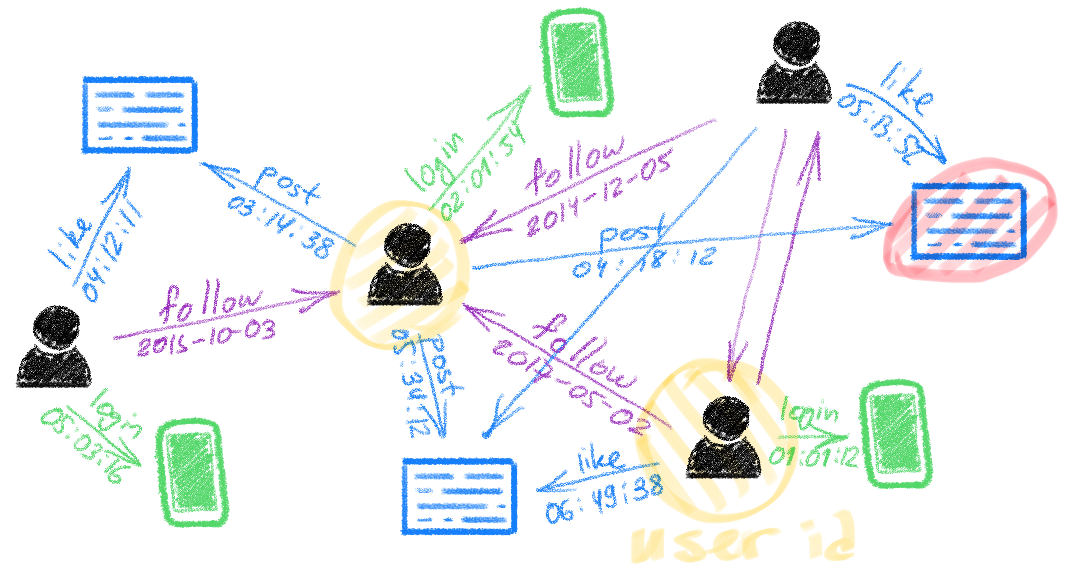Deep learning on graphs, also known as Geometric deep learning (GDL) , Graph representation learning (GRL), or relational inductive biases , has recently become one of the hottest topics in machine learning. While early works on graph learning go back at least a decade  if not two , it is undoubtedly the past few years’ progress that has taken these methods from a niche into the spotlight of the ML community and even to the popular science press (with Quanta Magazine running a series of excellent articles on geometric deep learning for the study of manifoldsdrug discovery, and protein science).

Graphs are powerful mathematical abstractions that can describe complex systems of relations and interactions in fields ranging from biology and high-energy physics to social science and economics. Since the amount of graph-structured data produced in some of these fields nowadays is enormous (prominent examples being social networks like Twitter and Facebook), it is very tempting to try to apply deep learning techniques that have been remarkably successful in other data-rich settings.

There are multiple flavours to graph learning problems that are largely application-dependent. One dichotomy is between node-wise and graph-wise problems, where in the former one tries to predict properties of individual nodes in the graph (e.g. identify malicious users in a social network), while in the latter one tries to make a prediction about the entire graph (e.g. predict solubility of a molecule). Furthermore, like in traditional ML problems, we can distinguish between supervised and unsupervised (or self-supervised) settings, as well as transductive and inductive problems.

Similarly to convolutional neural networks used in image analysis and computer vision, the key to efficient learning on graphs is designing local operations with shared weights that do message passing  between every node and its neighbours. A major difference compared to classical deep neural networks dealing with grid-structured data is that on graphs such operations are permutation-invariant, i.e. independent of the order of neighbour nodes, as there is usually no canonical way of ordering them.

Despite their promise and a series of success stories of graph representation learning (among which I can selfishly list the [acquisition by Twitter] of the graph-based fake news detection startup Fabula AI I have founded together with my students), we have not witnessed so far anything close to the smashing success convolutional networks have had in computer vision. In the following, I will try to outline my views on the possible reasons and how the field could progress in the next few years.

**Standardised benchmarks **like ImageNet were surely one of the key success factors of deep learning in computer vision, with some  even arguing that data was more important than algorithms for the deep learning revolution. We have nothing similar to ImageNet in scale and complexity in the graph learning community yet. The [Open Graph Benchmark] launched in 2019 is perhaps the first attempt toward this goal trying to introduce challenging graph learning tasks on interesting real-world graph-structured datasets. One of the hurdles is that tech companies producing diverse and rich graphs from their users’ activity are reluctant to share these data due to concerns over privacy laws such as GDPR. A notable exception is Twitter that made a dataset of 160 million tweets with corresponding user engagement graphs available to the research community under certain privacy-preserving restrictions as part of the [RecSys Challenge]. I hope that many companies will follow suit in the future.

**Software libraries **available in the public domain played a paramount role in “democratising” deep learning and making it a popular tool. If until recently, graph learning implementations were primarily a collection of poorly written and scarcely tested code, nowadays there are libraries such as [PyTorch Geometric] or [Deep Graph Library (DGL)] that are professionally written and maintained with the help of industry sponsorship. It is not uncommon to see an implementation of a new graph deep learning architecture weeks after it appears on arxiv.

Scalability is one of the key factors limiting industrial applications that often need to deal with very large graphs (think of Twitter social network with hundreds of millions of nodes and billions of edges) and low latency constraints. The academic research community has until recently almost ignored this aspect, with many models described in the literature completely inadequate for large-scale settings. Furthermore, graphics hardware (GPU), whose happy marriage with classical deep learning architectures was one of the primary forces driving their mutual success, is not necessarily the best fit for graph-structured data. In the long run, we might need specialised hardware for graphs .

**Dynamic graphs **are another aspect that is scarcely addressed in the literature. While graphs are a common way of modelling complex systems, such an abstraction is often too simplistic as real-world systems are dynamic and evolve in time. Sometimes it is the temporal behaviour that provides crucial insights about the system. Despite some recent progress, designing graph neural network models capable of efficiently dealing with continuous-time graphs represented as a stream of node- or edge-wise events is still an open research question.

#deep-learning #representation-learning #network-science #graph-neural-networks #geometric-deep-learning #deep learning1593529260

## Few Shot Learning — A Case Study (2)

In the previous blog, we looked into the fact why Few Shot Learning is essential and what are the applications of it. In this article, I will be explaining the Relation Network for Few-Shot Classification (especially for image classification) in the simplest way possible. Moreover, I will be analyzing the Relation Network in terms of:

1. Effectiveness of different architectures such as Residual and Inception Networks
2. Effects of transfer learning via using pre-trained classifier on ImageNet dataset

Moreover, effectiveness will be evaluated on the accuracy, time required for training, and the number of required training parameters.

Please watch the GitHub repository to check out the implementations and keep updated with further experiments.

# Introduction to Few-Shot Classification

In few shot classification, our objective is to design a method which can identify any object images by analyzing few sample images of the same class. Let’s the take one example to understand this. Suppose Bob has a client project to design a 5 class classifier, where 5 classes can be anything and these 5 classes can even change with time. As discussed in previous blog, collecting the huge amount of data is very tedious task. Hence, in such cases, Bob will rely upon few shot classification methods where his client can give few set of example images for each classes and after that his system can perform classification young these examples with or without the need of additional training.

In general, in few shot classification four terminologies (N way, K shot, support set, and query set) are used.

1. N way: It means that there will be total N classes which we will be using for training/testing, like 5 classes in above example.
2. K shot: Here, K means we have only K example images available for each classes during training/testing.
3. Support set: It represents a collection of all available K examples images from each classes. Therefore, in support set we have total N*K images.
4. Query set: This set will have all the images for which we want to predict the respective classes.

At this point, someone new to this concept will have doubt regarding the need of support and query set. So, let’s understand it intuitively. Whenever humans sees any object for the first time, we get the rough idea about that object. Now, in future if we see the same object second time then we will compare it with the image stored in memory from the when we see it for the first time. This applied to all of our surroundings things whether we see, read, or hear. Similarly, to recognise new images from query set, we will provide our model a set of examples i.e., support set to compare.

And this is the basic concept behind Relation Network as well. In next sections, I will be giving the rough idea behind Relation Network and I will be performing different experiments on 102-flower dataset.

The Core idea behind Relation Network is to learn the generalized image representations for each classes using support set such that we can compare lower dimensional representation of query images with each of the class representations. And based on this comparison decide the class of each query images. Relation Network has two modules which allows us to perform above two tasks:

1. Embedding module: This module will extract the required underlying representations from each input images irrespective of the their classes.
2. Relation Module: This module will score the relation of embedding of query image with each class embedding.

Training/Testing procedure:

We can define the whole procedure in just 5 steps.

1. Use the support set and get underlying representations of each images using embedding module.
2. Take the average of between each class images and get the single underlying representation for each class.
3. Then get the embedding for each query images and concatenate them with each class’ embedding.
4. Use the relation module to get the scores. And class with highest score will be the label of respective query image.
5. [Only during training] Use MSE loss functions to train both (embedding + relation) modules.Few things to know during the training is that we will use only images from the set of selective class, and during the testing, we will be using images from unseen classes. For example, from the 102-flower dataset, we will use 50% classes for training, and rest will be used for validation and testing. Moreover, in each episode, we will randomly select 5 classes to create the support and query set and follow the above 5 steps.

That is all need to know about the implementation point of view. Although the whole process is simple and easy to understand, I’ll recommend reading the published research paper, Learning to Compare: Relation Network for Few-Shot Learning, for better understanding.

#deep-learning #few-shot-learning #computer-vision #machine-learning #deep learning #deep learning